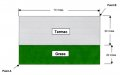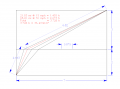# What is the fastest cycling route?

Thread Starter

#### Hymie

Joined Mar 30, 2018
963
The diagram below shows a section of a cycling course 70 miles by 50 miles (not to scale).

Each cyclist enters the section at the bottom left corner (point A) and must exit at the top right corner (point B), but otherwise may take any route between the two points.

As a cyclist in the race, you know that you can cycle at a constant speed of 30mph on tarmac, but only at half that speed (15mph) on grass. By taking the optimum route, how long will it take you to cycle from point A to point B?

(For the purposes of this question it is assumed that you enter at point A travelling at 15mph and in transitioning from the grass surface to tarmac, the time taken to accelerate from 15mph to 30mph is zero)#### MrChips

Joined Oct 2, 2009
23,077
I have the answer but is there an easy way without using calculus or a computer?

#### Ian Rogers

Joined Dec 12, 2012
840

#### WBahn

Joined Mar 31, 2012
26,398
This is just an disguised index of refraction problem in which the index of refraction of the tarmac is 1.0 and the index of refraction of the grass is 2.0.

I got approximately 3 hours, 43 minutes, and 36.41 seconds.

•boostbuck and jpanhalt

#### wayneh

Joined Sep 9, 2010
17,039
I have the answer but is there an easy way without using calculus or a computer?
I'm assuming Archimedes solved it by inspection.

#### Ian Rogers

Joined Dec 12, 2012
840
I actually got 3.7267 but rounded up wrong lol...

#### Ian Rogers

Joined Dec 12, 2012
840
I got approximately 3 hours, 43 minutes, and 36.41 seconds.
"approximately" Aw bless.... Sort of thing Spock would say!!

Thread Starter

#### Hymie

Joined Mar 30, 2018
963
I'm assuming Archimedes solved it by inspection.
Any method reaching the correct answer is allowed (including using the value of pi and the formula for the volume of a sphere)

#### MrChips

Joined Oct 2, 2009
23,077
Any method reaching the correct answer is allowed (including using the value of pi and the formula for the volume of a sphere)
In that case, the easiest method is to try all possible routes using a spread-sheet.
I did it using MATLAB.

#### jpanhalt

Joined Jan 18, 2008
11,088
Since I know almost no calculus, I turned to geometry (scale = 1:10). It was pretty obvious the initial path couldn't be 45°, so I calculated what looked about right.Then, I tried a little change off that point and got to 3h43'41" approximately. Is that close enough? I am embarrassed to have missed the refractive index part, but that has been almost 50 year in my past. Kudos to WBahn for that. Close, but not close enough for an Olympic race. Or, as wayneh said, by inspection.

John

PS: The tick marks are barely visible. They are at 1/8 distance of 70 miles.

#### WBahn

Joined Mar 31, 2012
26,398
"approximately" Aw bless.... Sort of thing Spock would say!!
That was because in his prior puzzles, Hymie has always insisted on an exact answer.

#### -live wire-

Joined Dec 22, 2017
912
What if you burn the grass down?

#### bogosort

Joined Sep 24, 2011
618
Here's an analytical solution, in case anyone is curious.

We recognize that the minimum-time solution will consist of two line segments that may or may not be colinear: first, a line segment from point A to some point C = (x, 20), followed by a line segment from C to the destination at point B. The total time is thus a function of a single variable x, which defines point C:

$$t(x) = \frac{d_1}{v_1} + \frac{d_2}{v_2}$$​

where d1 and d2 are the respective lengths of the two line segments, and v1 and v2 are the respective speeds.By inspection,

\begin{align*} d_1 &= \sqrt{x^2 + 20^2} d_2 &= \sqrt{(70 - x)^2 + 30^2} \end{align*}
and so

$$t(x) = \frac{\sqrt{x^2 + 20^2}}{15} + \frac{\sqrt{(70 - x)^2 + 30^2}}{30}$$​

To find the value of x that minimizes the function, we set its derivative to zero and solve for its root:

$$\frac{dt}{dx} = 0$$
With the gracious help of Wolfram Alpha,

$$\frac{x}{15 \sqrt{x^2 + 20^2}} - \frac{70 - x}{\sqrt{(70 - x)^2 + 30^2}} = 0 \Longrightarrow x = 10$$​

Plugging the root back into the total time function,

$$t(10) = \frac{\sqrt{10^2 + 20^2}}{15} + \frac{\sqrt{(70 - 10)^2 + 30^2}}{30} = 3.72678$$​

So, the path of shortest duration is when the cyclist rides a straight line to the 10 mile mark on the grass, and from there a straight line to the destination. The total trip time will be slightly less than 3.72678 hours (3 hours, 43 minutes, and 36.4 seconds), as WBahn calculated.

Using Snell's law, the angles the cyclist needs to ride can be easily found (first 26.57 degrees, followed by 63.43 degrees).

#### Attachments

•Chukman and wayneh

#### -live wire-

Joined Dec 22, 2017
912
You can't ignore the scenario where the biker burns the field down...

#### wayneh

Joined Sep 9, 2010
17,039
With the gracious help of Wolfram Alpha,

$$\frac{x}{15 \sqrt{x^2 + 20^2}} - \frac{70 - x}{\sqrt{(70 - x)^2 + 30^2}} = 0 \Longrightarrow x = 10$$​
I was contemplating doing this by hand and I was thinking it would probably be easier to solve for the minimum Time^2 instead of time. Should give the same answer. Didn't get around to it.

#### WBahn

Joined Mar 31, 2012
26,398
I was contemplating doing this by hand and I was thinking it would probably be easier to solve for the minimum Time^2 instead of time. Should give the same answer. Didn't get around to it.
The problem there is the cross-terms, so you still have a term that is the sum of two radicals.

#### wayneh

Joined Sep 9, 2010
17,039
The problem there is the cross-terms, so you still have a term that is the sum of two radicals.
Ah yes, of course. I would have seen that the second I tried to work it out. Thank goodness for Mathematica, or whatever it's become.

Thread Starter

#### Hymie

Joined Mar 30, 2018
963
Although the correct answer has been supplied in a number of posts – I don’t know how to solve this problem, or one with slightly modified parameters.

The solution I was given noted that the ratio of the sin of the two angles (ɵ1 & ɵ2 shown in bogosort’s post #13) is 2; and that this ratio is met with the cyclist riding to a 10 mile mark (on the grass) from the left hand edge. Although no explanation was given of how this location was determined.

I presume that if the cyclist could only manage 10 miles an hour on grass, then the sin ratio of the angles would be 3 (30 miles/hour vs 10 miles/hour).

I confirmed the given answer (with the transition from grass to tarmac at 10 miles) to be correct using an excel spreadsheet.

#### bogosort

Joined Sep 24, 2011
618
Although the correct answer has been supplied in a number of posts – I don’t know how to solve this problem, or one with slightly modified parameters.

The solution I was given noted that the ratio of the sin of the two angles (ɵ1 & ɵ2 shown in bogosort’s post #13) is 2; and that this ratio is met with the cyclist riding to a 10 mile mark (on the grass) from the left hand edge. Although no explanation was given of how this location was determined.
The value of 10 corresponds to the point where the total time function t(x) reaches its lowest value. In other words, graph t(x) as I described it above -- with the horizontal axis representing the mile marker crossed on the grass, and the vertical axis the total trip time -- then t(10) gives the lowest time. Analytically, this number is the root of t'(x), the first derivative of t(x).

I presume that if the cyclist could only manage 10 miles an hour on grass, then the sin ratio of the angles would be 3 (30 miles/hour vs 10 miles/hour).
Per the problem statement, the cyclist averages 15 mph on the grass (the number 10 in the 10-mile mark is the distance along the top edge of the grass, not a speed). Snell's law tells us that

\begin{align*} n_1 sin(\theta_1) &= n_2 sin(\theta_2) \frac{c}{v_1} sin(\theta_1) &= \frac{c}{v_2} sin(\theta_2) \frac{sin(\theta_1)}{v_1} &= \frac{sin(\theta_2)}{v_2} \end{align*}​

Therefore,

\begin{align*} \frac{sin(\theta_1)}{sin(\theta_2)} &= \frac{v_1}{v_2} &= \frac{15}{30} &= \frac{1}{2} \end{align*}
Looking at the implicit right triangles (and remembering SOHCAHTOA from trig class), we find that

$$\theta_1 = \arctan(10/20) \theta_2 = \arctan(60/30)$$​

and Snell's law is satisfied:

$$\frac{sin(\arctan(1/2))}{sin(\arctan(2))} = \frac{1}{2}$$​

Here's the geometry of the solution:The problem can easily be generalized by parametrizing the given dimensions and speeds in the formula for t(x).

#### WBahn

Joined Mar 31, 2012
26,398
Although the correct answer has been supplied in a number of posts – I don’t know how to solve this problem, or one with slightly modified parameters.

The solution I was given noted that the ratio of the sin of the two angles (ɵ1 & ɵ2 shown in bogosort’s post #13) is 2; and that this ratio is met with the cyclist riding to a 10 mile mark (on the grass) from the left hand edge. Although no explanation was given of how this location was determined.

I presume that if the cyclist could only manage 10 miles an hour on grass, then the sin ratio of the angles would be 3 (30 miles/hour vs 10 miles/hour).

I confirmed the given answer (with the transition from grass to tarmac at 10 miles) to be correct using an excel spreadsheet.
The basic notion of the solution is that there are two competing factors. You want to spend as little time on the grass as possible and you want to keep the total distance as short as possible. If you draw a straight line from start to finish, you have the shortest overall distance, but you have a relatively long distance on the grass. If you head vertically to the top of the grass and then head diagonally to the end, you have the shortest distance on grass, but a relatively long distance overall. So there is a point along the grass/tarmac boundary somewhere between these two that results in the best compromise.

To solve the problem of where that point is, you parameterize it in terms of the location along the boundary and set up an equation for the total time to travel along the resulting path. You then take the derivative with respect to time and set it equal to zero to fine the minima. In rectangular coordinates the result is a mess. But by performing trig substitutions you can parameterize the equation according to the angles between the two tracks and the normal line at the boundary and the problem becomes quite tractable. The result is Snell's Law, which says that the desired point on the boundary is located where the ratio of the sines of the two angles is equal to the ratio of the velocities in the respective media.

•MrChips